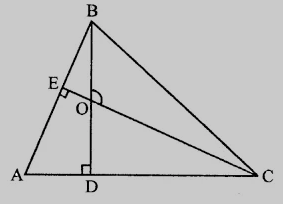# In $\triangle ABC, BD \perp AC$ and $CE \perp AB$. If $BD$ and $CE$ intersect at $O$, prove that $\angle BOC = 180^o-\angle A$.

Given:

In $\triangle ABC, BD \perp AC$ and $CE \perp AB$.

$BD$ and $CE$ intersect at $O$.

To do:

We have to prove that $\angle BOC = 180^o-\angle A$.

Solution:From the figure,

In quadrilateral $ADOE$,

$\angle A + \angle D + \angle O + \angle E = 360° (Sum of angles of quadrilateral)$\angle A + 90^o + \angle DOE + 90^o = 360^o\angle A + \angle DOE = 360^o - 180^o = 180^o\angle BOC = \angle DOE$(Vertically opposite angles) This implies,$\angle A + \angle BOC = 180^o\angle BOC = 180^o - \angle A\$

Hence proved.

Updated on: 10-Oct-2022

29 Views